International
Tables for
Crystallography
Volume F
Crystallography of biological macromolecues
Edited by M. G. Rossmann and E. Arnold

International Tables for Crystallography (2006). Vol. F, ch. 22.3, pp. 555-556   | 1 | 2 |

## Section 22.3.3. Applications

K. A. Sharpa*

aE. R. Johnson Research Foundation, Department of Biochemistry and Biophysics, University of Pennsylvania, Philadelphia, PA 19104-6059, USA
Correspondence e-mail: sharp@crystal.med.upenn.edu

### 22.3.3. Applications

| top | pdf |

An exhaustive list of applications of classical electrostatic modelling to macromolecules is beyond the scope of this chapter. Three general areas of application are discussed.

#### 22.3.3.1. Electrostatic potential distributions

| top | pdf |

Graphical analysis of electrostatic potential distributions often reveals features about the structure that complement analysis of the atomic coordinates. For example, Fig. 22.3.3.1(a)shows the distribution of charged residues in the binding site of the proteolytic enzyme thrombin. Fig. 22.3.3.1(b)shows the resulting electrostatic potential distribution on the protein surface. The basic (positive) region in the fibrinogen binding site, which could be inferred from close inspection of the distribution of charged residues in Fig. 22.3.3.1(a), is clearly more apparent in the potential distribution. Fig. 22.3.3.1(c)shows the effect of increasing ionic strength on the potential distribution, shrinking the regions of strong potential. Fig. 22.3.3.1(d)is calculated assuming the same dielectric for the solvent and protein. The more uniform potential distribution compared to Fig. 22.3.3.1(b)shows the focusing effect that the low dielectric interior has on the field emanating from charges in active sites and other cleft regions.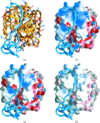Figure 22.3.3.1 | top | pdf |(a) The proteolytic enzyme thrombin (yellow backbone worm) complexed with an inhibitor, hirudin (blue backbone worm). The negatively charged (red) and positively charged (blue) side chains of thrombin are shown in bond representation. (b) Solvent-accessible surface of thrombin coded by electrostatic potential (blue: positive, red: negative). Hirudin is shown as a blue backbone worm. Potential is calculated at zero ionic strength. (c) Solvent-accessible surface of thrombin coded by electrostatic potential (blue: positive, red: negative). Hirudin is shown as a blue backbone worm. Potential is calculated at physiological ionic strength (0.145 M). (d) Solvent-accessible surface of thrombin coded by electrostatic potential (blue: positive, red: negative). Hirudin is shown as a blue backbone worm. Potential is calculated using the same polarizability for protein and solvent.

#### 22.3.3.2. Charge-transfer equilibria

| top | pdf |

Charge-transfer processes are important in protein catalysis, binding, conformational changes and many other functions. The primary examples are acid–base equilibria, electron transfer and ion binding, in which the transferred species is a proton, an electron or a salt ion, respectively. The theory of the dependence of these three equilibria within the classical electrostatic framework can be treated in an identical manner, and will be illustrated with acid–base equilibria. A titratable group will have an intrinsic ionization equilibrium, expressed in terms of a known intrinsic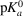, where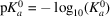,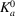is the dissociation constant for the reaction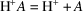and A can be an acid or a base. Theis determined by all the quantum-chemical, electrostatic and environmental effects operating on that group in some reference state. For example, a reference state for the aspartic acid side-chain ionization might be the isolated amino acid in water, for which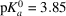. In the environment of the protein, the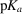will be altered by three electrostatic effects. The first occurs because the group is positioned in a protein environment with a different polarizability, the second is due to interaction with permanent dipoles in the protein, the third is due to charged, perhaps titratable, groups. The effectiveis given by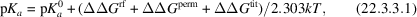where the factor of 1/2.303kT converts units of energy to units of. The first contribution,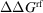, arises because the completely solvated group induces a strong favourable reaction field (see Section 22.3.2.3) in the high dielectric water, which stabilizes the charged form of the group. (The neutral form is also stabilized by the solvent reaction field induced by any dipolar groups, but to a lesser extent.) Desolvating the group to any degree by moving it into a less polarizable environment will preferentially destabilize the charged form of that group, shifting theby an amount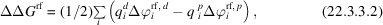where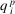and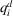are the charge distributions on the group,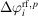and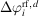are the changes in the group's reaction potential upon moving it from its reference state into the protein, in the protonated (superscript p) and deprotonated (superscript d) forms, respectively, and the sum is over the group's charges. The contribution of the permanent dipoles is given by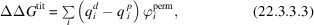where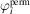is the interaction potential at the ith charge due to all the permanent dipoles in the protein, including the effect of screening. It is observed that intrinsic's of groups in proteins are rarely shifted by more than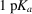unit, indicating that the effects of desolvation are often compensated to a large degree by the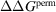term (Antosiewicz et al., 1994). The final term accounts for the contribution of all the other charged groups: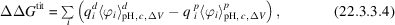where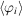is the mean potential at group charge i from all the other titratable groups. The charge states of the other groups in the protein depend in turn on their intrinsic `'s', on the external pH if they are acid–base groups, the external redox potential,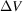, if they are redox groups and the concentration of ions, c, if they are ion-binding sites, as indicated by the subscript to. Moreover, the charge state of the group itself will affect the equilibrium at the other sites. Because of this linkage, exact determination of the complete charged state of a protein is a complex procedure. If there are N such groups, the rigorous approach is to compute the titration-state partition function by evaluating the relative electrostatic free energies of all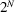ionization states for a given set of pH, c,. From this one may calculate the mean ionization state of any group as a function of pH,etc. For large N this becomes impractical, but various approximate schemes work well, including a Monte Carlo procedure (Beroza et al., 1991; Yang et al., 1993) or partial evaluation of the titration partition function by clustering the groups into strongly interacting sub-domains (Bashford & Karplus, 1990; Gilson, 1993; Yang et al., 1993).

Calculation of ion-binding and electron-transfer equilibria in proteins proceeds exactly as for calculation of acid–base equilibria, the results usually being expressed in terms of an association constant,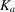, or a redox midpoint potential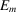(defined as the external reducing potential at which the group is half oxidized and half reduced, usually at pH 7), respectively.

#### 22.3.3.3. Electrostatic contributions to binding energy

| top | pdf |

The electrostatic contribution to the binding energy of two molecules is obtained by taking the difference in total electrostatic energies in the bound (AB) and unbound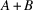states. For the linear case,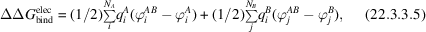where the first and second sums are over all charges in molecule A and B, respectively, and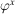is the total potential produced by x = A, B, or AB. From equation (22.3.3.5), it should be noted that the electrostatic free energy change of each molecule has contributions from intermolecular charge–charge interactions, and from changes in the solvent reaction potential of the molecule itself when solvent is displaced by the other molecule. Equation (22.3.3.5) allows for the possibility that the conformation may change upon binding, since different charge distributions may be used for the complexed and uncomplexed forms of A, and similarly for B. However, other energetic terms, including those involved in any conformational change, have to be added to equation (22.3.3.5) to obtain net binding free energy changes. Nevertheless, changes in binding free energy due to charge modifications or changes in external factors such as pH and salt concentration may be estimated using equation (22.3.3.5) alone. For the latter, salt effects are usually only significant in highly charged molecules, for which the nonlinear form for the total electrostatic energy, equation (22.3.2.4), must be used. The salt dependence of binding of drugs and proteins to DNA has been studied using this approach (Misra, Hecht et al., 1994; Misra, Sharp et al., 1994; Sharp et al., 1995), including the pH dependence of drug binding (Misra & Honig, 1995). Other applications include the binding of sulfate to the sulfate binding protein (Åqvist et al., 1991) and antibody and antigen interactions (Lee et al., 1992; Slagle et al., 1994).

### References

Antosiewicz, J., McCammon, J. A. & Gilson, M. K. (1994). Prediction of pH-dependent properties of proteins. J. Mol. Biol. 238, 415–436.Google Scholar
Bashford, D. & Karplus, M. (1990). pKa's of ionizable groups in proteins: atomic detail from a continuum electrostatic model. Biochemistry, 29, 10219–10225.Google Scholar
Beroza, P., Fredkin, D., Okamura, M. & Feher, G. (1991). Protonation of interacting residues in a protein by a Monte-Carlo method. Proc. Natl Acad. Sci. USA, 88, 5804–5808.Google Scholar
Gilson, M. (1993). Multiple-site titration and molecular modeling: 2. Rapid methods for computing energies and forces for ionizable groups in proteins. Proteins Struct. Funct. Genet. 15, 266–282.Google Scholar
Lee, F. S., Chu, Z. T., Bolger, M. B. & Warshel, A. (1992). Calculations of antibody–antigen interactions: microscopic and semi-microscopic evaluation of the free energies of binding of phosphorylcholine analogs to Mcpc603. Protein Eng. 5, 215–228.Google Scholar
Misra, V., Hecht, J., Sharp, K., Friedman, R. & Honig, B. (1994). Salt effects on protein–DNA interactions: the lambda cI repressor and Eco R1 endonuclease. J. Mol. Biol. 238, 264–280.Google Scholar
Misra, V. & Honig, B. (1995). On the magnitude of the electrostatic contribution to ligand–DNA interactions. Proc. Natl Acad. Sci. USA, 92, 4691–4695.Google Scholar
Misra, V., Sharp, K., Friedman, R. & Honig, B. (1994). Salt effects on ligand–DNA binding: minor groove antibiotics. J. Mol. Biol. 238, 245–263.Google Scholar
Sharp, K. A., Friedman, R., Misra, V., Hecht, J. & Honig, B. (1995). Salt effects on polyelectrolyte–ligand binding: comparison of Poisson–Boltzmann and limiting law counterion binding models. Biopolymers, 36, 245–262.Google Scholar
Slagle, S., Kozack, R. E. & Subramaniam, S. (1994). Role of electrostatics in antibody–antigen association: anti-hen egg lysozyme/lysozyme complex (HyHEL–5/HEL). J. Biomol. Struct. Dyn. 12, 439–456.Google Scholar
Yang, A., Gunner, M., Sampogna, R., Sharp, K. & Honig, B. (1993). On the calculation of pKa in proteins. Proteins Struct. Funct. Genet. 15, 252–265.Google Scholar
Åqvist, J., Luecke, H., Quiocho, F. A. & Warshel, A. (1991). Dipoles localized at helix termini of proteins stabilize charges. Proc. Natl Acad. Sci. USA, 88, 2026–2030.Google Scholar